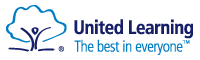# Exam Outcomes, DfE Performance Tables and Destinations

### Exam Results 2021-22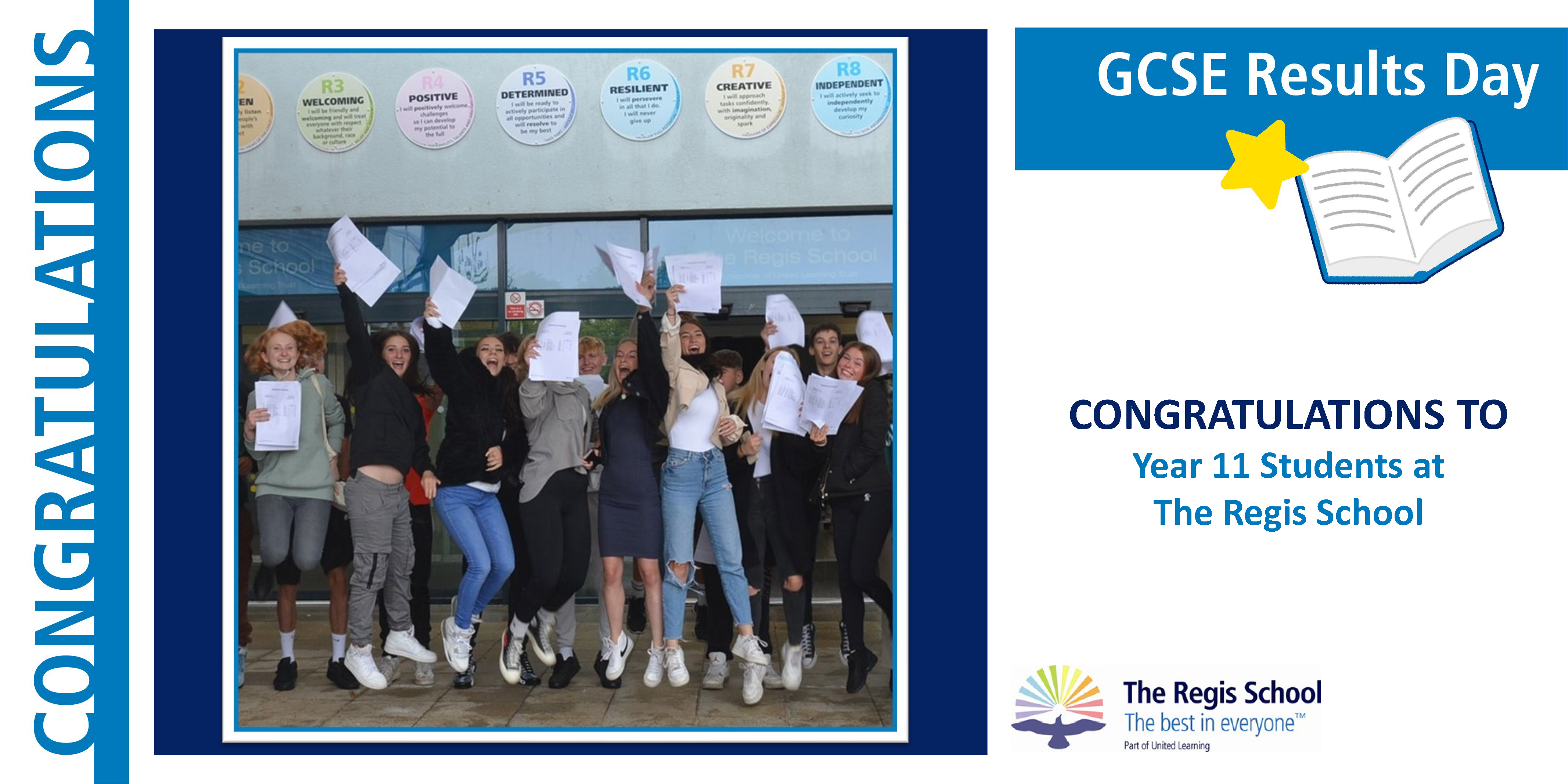GCSE

Progress 8 score = +0.53

Attainment 8 score = 47.15

Basics 4+ = 70%

Basics 5+ = 54%

Percentage of students who entered the EBacc = 41%

Percentage of students who achieved the EBacc at 5+ = 15%

Level 3 (A levels and BTECs)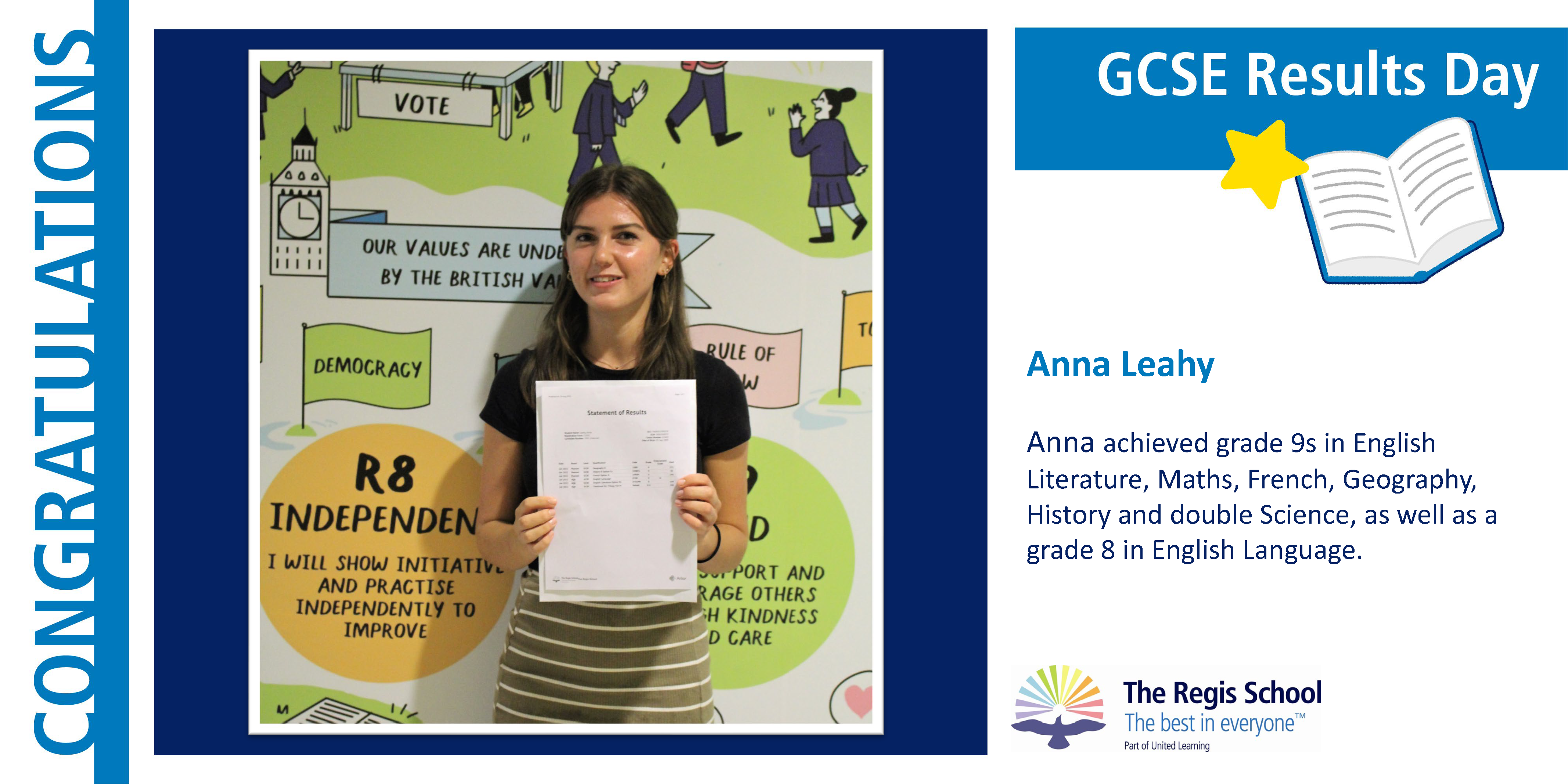A Level

Percentage of grades at A*-B = 66.39%

Percentage of grades at A*-C = 88.24%

Overall pass rate (A*-E) = 100.0%

BTEC/Applied General

Percentage of grades at Distinction* - Distinction = 84%

Percentage of grades at Distinction* - Merit = 97%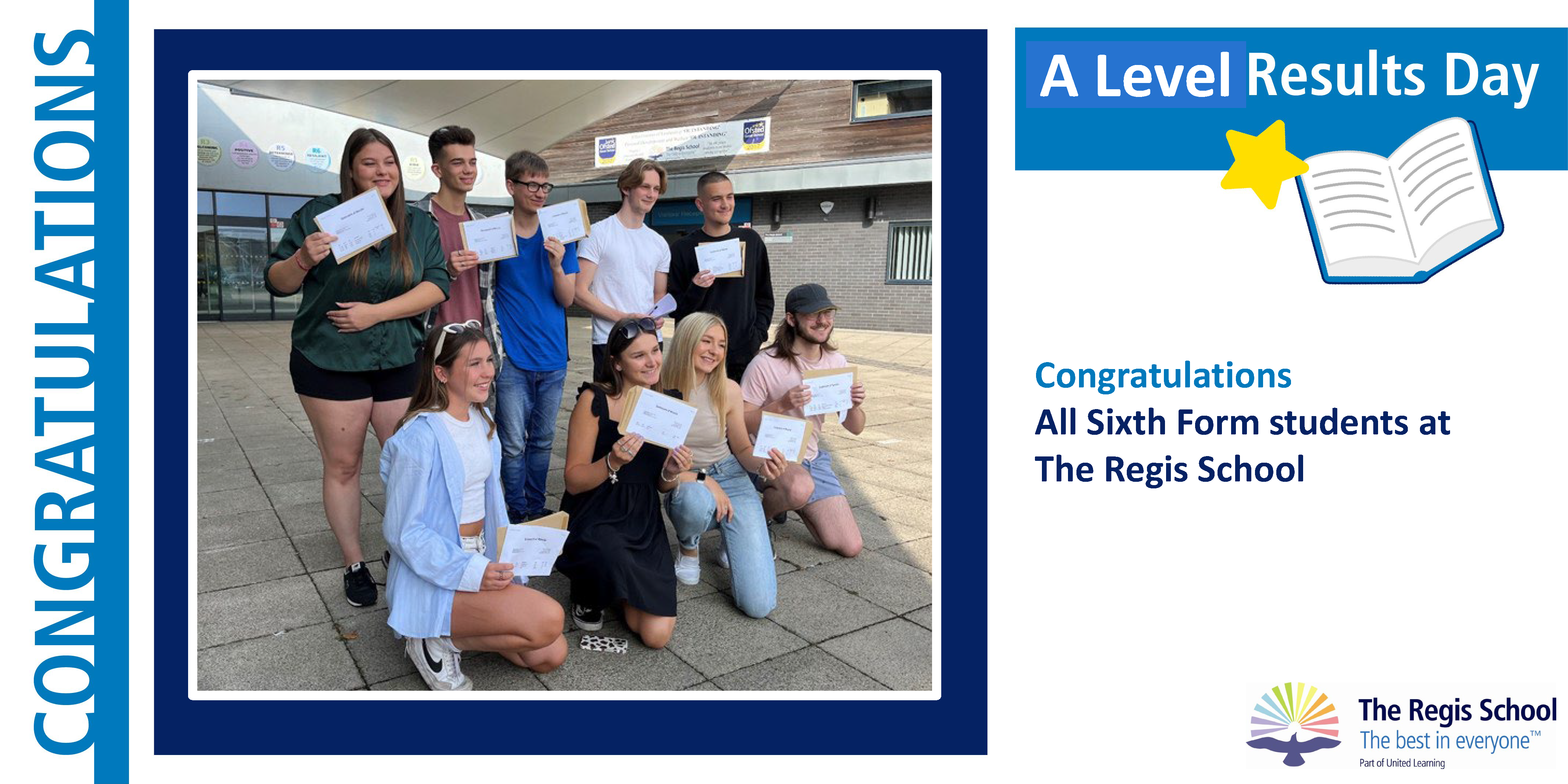Percentage of grades A*-B = 50%

Percentage of grades A*-C = 75%

Overall pass rate (D*-P/A*-E) = 100.0%

### Exam Results 2018 - 19

Please note these are not our latest set of results as due to the Coronavirus pandemic the results for 2019-20 were 'Awarded Grades' and results for 2020-21 were 'Teacher Assessed Grades' and they therefore have not been published as performance measures by the Secretary of State.

GCSE

Progress 8 score = -0.05

Attainment 8 score = 44.65Basics 4+ = 62%

Basics 5+ = 35%

Percentage of students who entered the EBacc = 37%

Percentage of students who achieved the EBacc at 5+ = 10%

Level 3 (A levels and BTECs)

A Level

Percentage of grades at A*-B = 43%

Percentage of grades at A*-C = 69%

Overall pass rate (A*-E) = 98%

BTEC

Percentage of grades at Distinction* - Distinction = 80%

Percentage of grades at Distinction* - Merit = 95%

Overall pass rate (D*-P) = 100%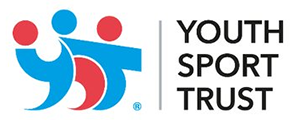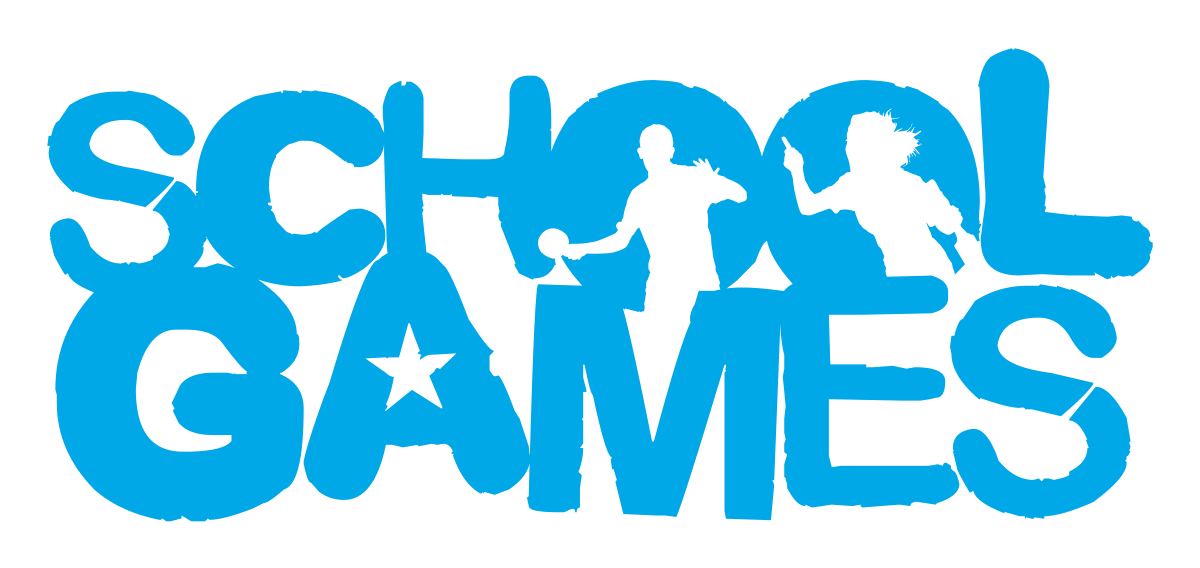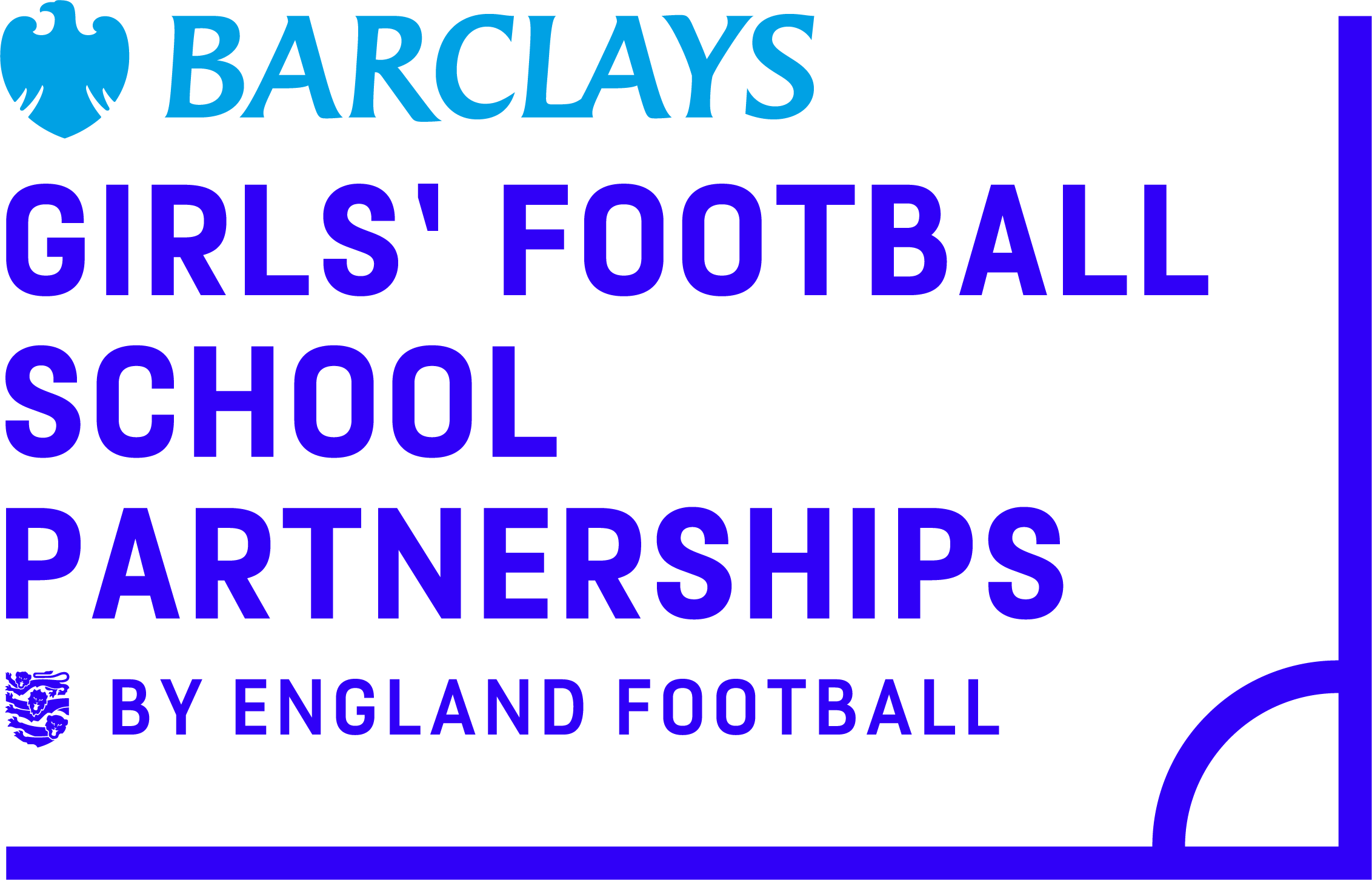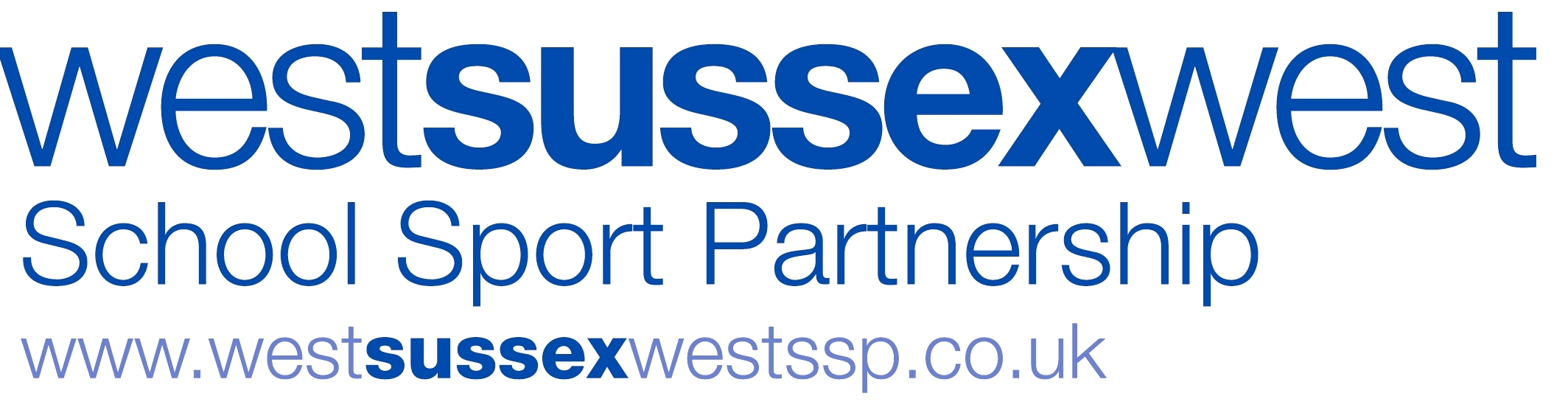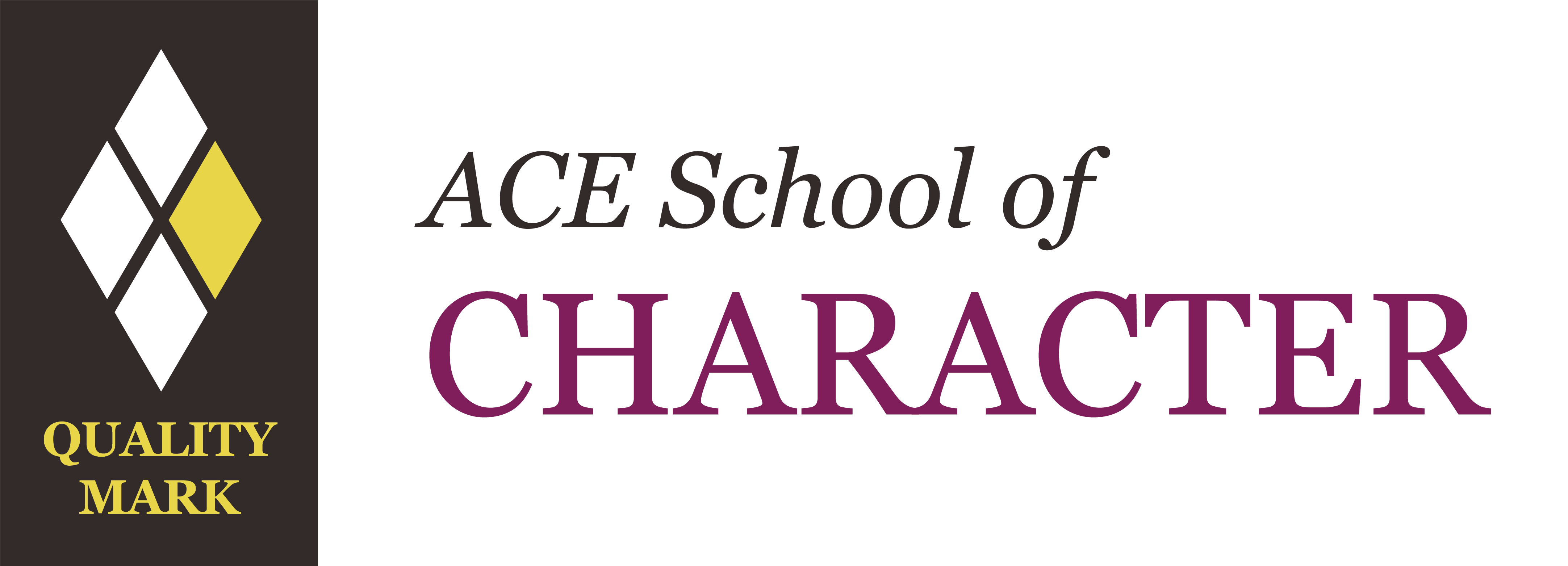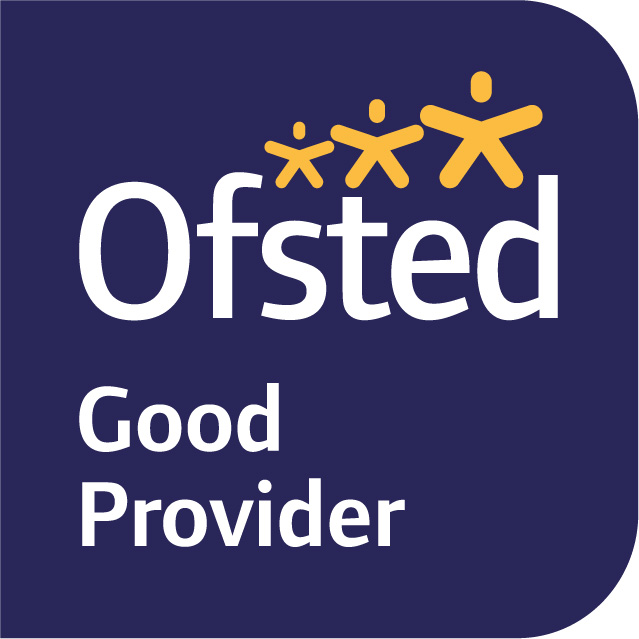The school is part of United Learning. United Learning comprises: UCST (Registered in England No: 2780748. Charity No. 1016538) and ULT (Registered in England No. 4439859. An Exempt Charity). Companies limited by guarantee. VAT number 834 8515 12.
Registered address: United Learning, Worldwide House, Thorpe Wood, Peterborough, PE3 6SB. Tel: 01832 864 444

Financial Accountability and Freedom of Information